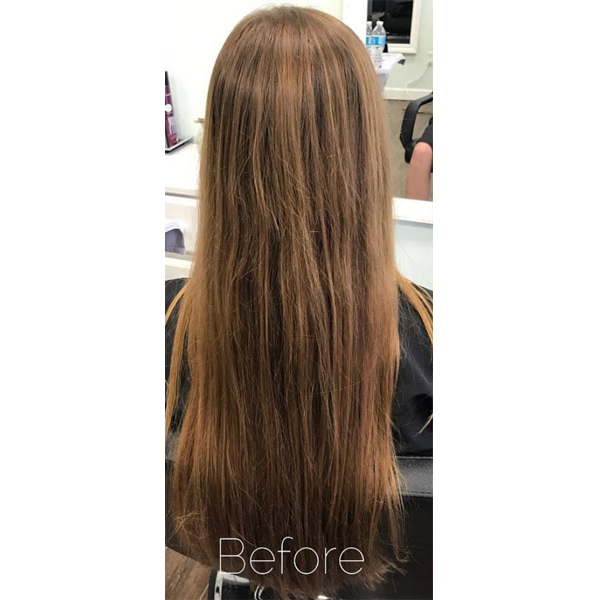Journey To Dimensional Blonde
Last updated: November 08, 2018

# Journey To Dimensional Blonde• Natural/Starting Level:

Vibrant Red

• First Session

Formula A: Equal parts PRAVANA Artificial Hair Color Extractor Step 1 + Step 2

Formula B: Equal parts Matrix Color Sync 8N + 8P + 10-volume developer

• Second Session

Formula A (balayage): Kenra Professional Simply Blonde Clay Lightener + b3 Brazilian Bond Builder

Formula B (toner): Equal parts Redken Shades EQ 9T + 9P + Shades EQ Processing Solution

• Third Session

Formula A: Matrix Light Master + 30-volume developer + b3 Brazilian Bond Builder

Formula B: Equal parts Matrix Color Sync Demi 10V + 10-volume developer

Formula C: Equal parts Matrix Color Sync Demi SPV + 10-volume developer

No two transformations are the same. Sometimes your client has the most beautiful honey blonde base, making color application swift, easy and a one-day appointment. Other times, the long-time vibrant redhead in your chair decides she’s ready to try a dimensional blonde shade, meaning it’ll take multiple sessions to get it done. No worries!

When Mallery Share (@hellobalayage), owner of Simplicity Salon in Port St. Lucie, Fla., found herself in this situation, she welcomed the challenge of a major transformation. Knowing such a big change would require time, Mallery broke the transformation into three sessions—remove the red then apply the balayage then brighten the balayage. Find out how she accomplished the transformation!

Total Processing Time: 9 hours (3 hours per session) over a 10 week time period.

Pricing: Each session ranged from \$200 to \$250, so the total cost of the transformation ranged from \$600 to \$750.

## COLOR FORMULAS• Natural/Starting Level:

Vibrant Red

• First Session

Formula A: Equal parts PRAVANA Artificial Hair Color Extractor Step 1 + Step 2

Formula B: Equal parts Matrix Color Sync 8N + 8P + 10-volume developer

• Second Session

Formula A (balayage): Kenra Professional Simply Blonde Clay Lightener + b3 Brazilian Bond Builder

Formula B (toner): Equal parts Redken Shades EQ 9T + 9P + Shades EQ Processing Solution

• Third Session

Formula A: Matrix Light Master + 30-volume developer + b3 Brazilian Bond Builder

Formula B: Equal parts Matrix Color Sync Demi 10V + 10-volume developer

Formula C: Equal parts Matrix Color Sync Demi SPV + 10-volume developer

## HOW-TO STEPS•• Natural/Starting Level:

Vibrant Red

• First Session

Formula A: Equal parts PRAVANA Artificial Hair Color Extractor Step 1 + Step 2

Formula B: Equal parts Matrix Color Sync 8N + 8P + 10-volume developer

• Second Session

Formula A (balayage): Kenra Professional Simply Blonde Clay Lightener + b3 Brazilian Bond Builder

Formula B (toner): Equal parts Redken Shades EQ 9T + 9P + Shades EQ Processing Solution

• Third Session

Formula A: Matrix Light Master + 30-volume developer + b3 Brazilian Bond Builder

Formula B: Equal parts Matrix Color Sync Demi 10V + 10-volume developer

Formula C: Equal parts Matrix Color Sync Demi SPV + 10-volume developer

1

First Session

1. Similar to applying color at the bowl, saturate the color you wish to remove by applying Formula A with a brush. “This will not adjust or alter her natural haircolor,” notes Mallery.

2. Then, place the hair in a plastic cap and process for 20 minutes under a dryer.

3. Clarify the hair again, then apply 10-volume developer to ensure the color was removed. Repeat the process. “This left the hair very soft without any damage and helped remove most of the red she had for years,” shares Mallery.

4. To take her client’s hair back to a natural color, Mallery applied Formula B and let process for 15 minutes.

•• Natural/Starting Level:

Vibrant Red

• First Session

Formula A: Equal parts PRAVANA Artificial Hair Color Extractor Step 1 + Step 2

Formula B: Equal parts Matrix Color Sync 8N + 8P + 10-volume developer

• Second Session

Formula A (balayage): Kenra Professional Simply Blonde Clay Lightener + b3 Brazilian Bond Builder

Formula B (toner): Equal parts Redken Shades EQ 9T + 9P + Shades EQ Processing Solution

• Third Session

Formula A: Matrix Light Master + 30-volume developer + b3 Brazilian Bond Builder

Formula B: Equal parts Matrix Color Sync Demi 10V + 10-volume developer

Formula C: Equal parts Matrix Color Sync Demi SPV + 10-volume developer

2

Second Session

1. Balayage Formula A in a V-pattern, saturating the ends. Leave little hair between sections and place a foil on top to lock in moisture and ensure the highest lift possible.

2. At the crown, babylight balayage Formula A for a piecey finish.

3. Process for 45 minutes, then shampoo and condition.

4. Towel-dry, then tone using Formula B and process for 20 minutes.

5. Blow-dry, then curl the hair using a 1¼-inch BaByliss Pro curling iron.

•• Natural/Starting Level:

Vibrant Red

• First Session

Formula A: Equal parts PRAVANA Artificial Hair Color Extractor Step 1 + Step 2

Formula B: Equal parts Matrix Color Sync 8N + 8P + 10-volume developer

• Second Session

Formula A (balayage): Kenra Professional Simply Blonde Clay Lightener + b3 Brazilian Bond Builder

Formula B (toner): Equal parts Redken Shades EQ 9T + 9P + Shades EQ Processing Solution

• Third Session

Formula A: Matrix Light Master + 30-volume developer + b3 Brazilian Bond Builder

Formula B: Equal parts Matrix Color Sync Demi 10V + 10-volume developer

Formula C: Equal parts Matrix Color Sync Demi SPV + 10-volume developer

3

Third Session

1. Repeat the same process followed in the second session, but note the change in Formulas A and B.

2. Then, apply Formula C from the midlenthgs to ends and process for 20 minutes.

3. Blow-dry the hair then smooth her stands using a flat iron.